Next: Quantum statistics in the Up: Quantum statistics Previous: Bose-Einstein statistics

## Maxwell-Boltzmann statistics

For the purpose of comparison, it is instructive to consider the purely classical case of Maxwell-Boltzmann statistics. The partition function is written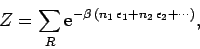(609)

where the sum is over all distinct statesof the gas, and the particles are treated as distinguishable. For given values ofthere are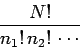(610)

possible ways in whichdistinguishable particles can be put into individual quantum states such that there are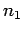particles in state 1,particles in state 2, etc. Each of these possible arrangements corresponds to a distinct state for the whole gas. Hence, Eq. (609) can be written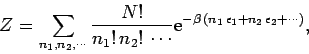(611)

where the sum is over all values of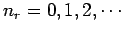for each, subject to the constraint that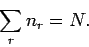(612)

Now, Eq. (611) can be written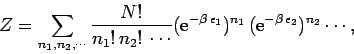(613)

which, by virtue of Eq. (612), is just the result of expanding a polynomial. In fact,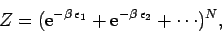(614)

or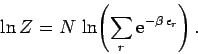(615)

Note that the argument of the logarithm is simply the partition function for a single particle.

Equations (583) and (615) can be combined to give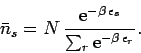(616)

This is known as the Maxwell-Boltzmann distribution. It is, of course, just the result obtained by applying the Boltzmann distribution to a single particle (see Sect. 7).Next: Quantum statistics in the Up: Quantum statistics Previous: Bose-Einstein statistics
Richard Fitzpatrick 2006-02-02